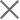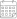Subscribe

### Indexes

June 2023 | Electricity Market Review27 July 2023

In June 2023, Georgian power plants generated 1,369 mln. kWh of electricity (Figure 1). This represents an 8% decrease in the total generation compared to the previous year (in June 2022, the total generation was 1,485 mln. kWh). The decrease in generation on a yearly basis comes from a fall of 8% in Hydro and 9% in wind and 46% in thermal power generation.

On a monthly basis, the generation increased by approximately 3% (in May 2023, the total generation was 1,325 mln. kWh) (Figure 1). The monthly rise in total generation is induced by a 3% increase in hydro and 104% in thermal power generation, while wind power generation decreased by 30%.

The consumption of electricity on the local market was 981 mln. kWh (-9% compared to June 2022, and -5% compared to May 2023) (Figure 1). In June 2023, power generation exceeded consumption by 387 mln. kWh which was 28% of the total generation and 39% of the total consumption (in June 2022, the difference between the total generation and the consumption resulted in a surplus of 403 mln. kWh, around 27% of the total generation and 37% of the total consumption for the month).